# Stable Diffusion with 🧨 Diffusers# Stable Diffusion 🎨

...using 🧨 Diffusers

Stable Diffusion is a text-to-image latent diffusion model created by the researchers and engineers from CompVis, Stability AI and LAION. It is trained on 512x512 images from a subset of the LAION-5B database. LAION-5B is the largest, freely accessible multi-modal dataset that currently exists.

In this post, we want to show how to use Stable Diffusion with the 🧨 Diffusers library, explain how the model works and finally dive a bit deeper into how diffusers allows one to customize the image generation pipeline.

Note: It is highly recommended to have a basic understanding of how diffusion models work. If diffusion models are completely new to you, we recommend reading one of the following blog posts:

Now, let's get started by generating some images 🎨.

## Running Stable Diffusion

Before using the model, you need to accept the model license in order to download and use the weights. Note: the license does not need to be explicitly accepted through the UI anymore.

The license is designed to mitigate the potential harmful effects of such a powerful machine learning system. We request users to read the license entirely and carefully. Here we offer a summary:

1. You can't use the model to deliberately produce nor share illegal or harmful outputs or content,
2. We claim no rights on the outputs you generate, you are free to use them and are accountable for their use which should not go against the provisions set in the license, and
3. You may re-distribute the weights and use the model commercially and/or as a service. If you do, please be aware you have to include the same use restrictions as the ones in the license and share a copy of the CreativeML OpenRAIL-M to all your users.

### Usage

First, you should install diffusers==0.10.2 to run the following code snippets:

pip install diffusers==0.10.2 transformers scipy ftfy accelerate


In this post we'll use model version v1-4, but you can also use other versions of the model such as 1.5, 2, and 2.1 with minimal code changes.

The Stable Diffusion model can be run in inference with just a couple of lines using the StableDiffusionPipeline pipeline. The pipeline sets up everything you need to generate images from text with a simple from_pretrained function call.

from diffusers import StableDiffusionPipeline

pipe = StableDiffusionPipeline.from_pretrained("CompVis/stable-diffusion-v1-4")


If a GPU is available, let's move it to one!

pipe.to("cuda")


Note: If you are limited by GPU memory and have less than 10GB of GPU RAM available, please make sure to load the StableDiffusionPipeline in float16 precision instead of the default float32 precision as done above.

You can do so by loading the weights from the fp16 branch and by telling diffusers to expect the weights to be in float16 precision:

import torch
from diffusers import StableDiffusionPipeline

pipe = StableDiffusionPipeline.from_pretrained("CompVis/stable-diffusion-v1-4", revision="fp16", torch_dtype=torch.float16)


To run the pipeline, simply define the prompt and call pipe.

prompt = "a photograph of an astronaut riding a horse"

image = pipe(prompt).images

# you can save the image with
# image.save(f"astronaut_rides_horse.png")


The result would look as follows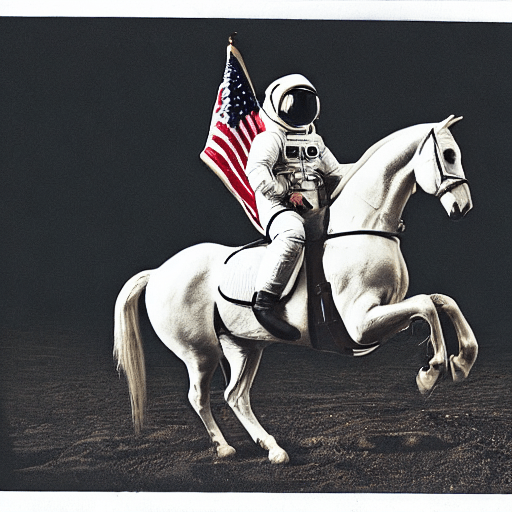The previous code will give you a different image every time you run it.

If at some point you get a black image, it may be because the content filter built inside the model might have detected an NSFW result. If you believe this shouldn't be the case, try tweaking your prompt or using a different seed. In fact, the model predictions include information about whether NSFW was detected for a particular result. Let's see what they look like:

result = pipe(prompt)
print(result)

{
'images': [<PIL.Image.Image image mode=RGB size=512x512>],
'nsfw_content_detected': [False]
}


If you want deterministic output you can seed a random seed and pass a generator to the pipeline. Every time you use a generator with the same seed you'll get the same image output.

import torch

generator = torch.Generator("cuda").manual_seed(1024)
image = pipe(prompt, guidance_scale=7.5, generator=generator).images

# you can save the image with
# image.save(f"astronaut_rides_horse.png")


The result would look as follows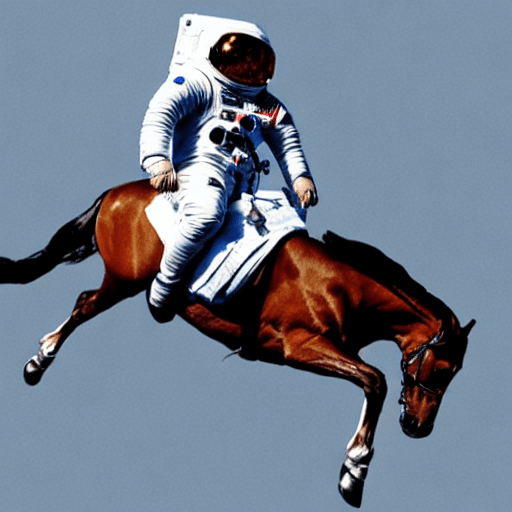You can change the number of inference steps using the num_inference_steps argument.

In general, results are better the more steps you use, however the more steps, the longer the generation takes. Stable Diffusion works quite well with a relatively small number of steps, so we recommend to use the default number of inference steps of 50. If you want faster results you can use a smaller number. If you want potentially higher quality results, you can use larger numbers.

Let's try out running the pipeline with less denoising steps.

import torch

generator = torch.Generator("cuda").manual_seed(1024)
image = pipe(prompt, guidance_scale=7.5, num_inference_steps=15, generator=generator).images

# you can save the image with
# image.save(f"astronaut_rides_horse.png")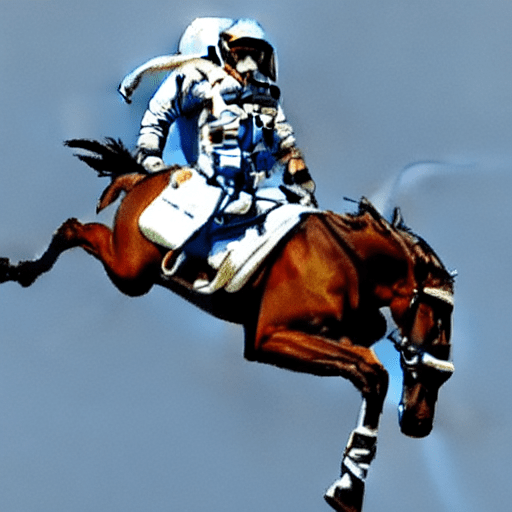Note how the structure is the same, but there are problems in the astronauts suit and the general form of the horse. This shows that using only 15 denoising steps has significantly degraded the quality of the generation result. As stated earlier 50 denoising steps is usually sufficient to generate high-quality images.

Besides num_inference_steps, we've been using another function argument, called guidance_scale in all previous examples. guidance_scale is a way to increase the adherence to the conditional signal that guides the generation (text, in this case) as well as overall sample quality. It is also known as classifier-free guidance, which in simple terms forces the generation to better match the prompt potentially at the cost of image quality or diversity. Values between 7 and 8.5 are usually good choices for Stable Diffusion. By default the pipeline uses a guidance_scale of 7.5.

If you use a very large value the images might look good, but will be less diverse. You can learn about the technical details of this parameter in this section of the post.

Next, let's see how you can generate several images of the same prompt at once. First, we'll create an image_grid function to help us visualize them nicely in a grid.

from PIL import Image

def image_grid(imgs, rows, cols):
assert len(imgs) == rows*cols

w, h = imgs.size
grid = Image.new('RGB', size=(cols*w, rows*h))
grid_w, grid_h = grid.size

for i, img in enumerate(imgs):
grid.paste(img, box=(i%cols*w, i//cols*h))
return grid


We can generate multiple images for the same prompt by simply using a list with the same prompt repeated several times. We'll send the list to the pipeline instead of the string we used before.

num_images = 3
prompt = ["a photograph of an astronaut riding a horse"] * num_images

images = pipe(prompt).images

grid = image_grid(images, rows=1, cols=3)

# you can save the grid with
# grid.save(f"astronaut_rides_horse.png")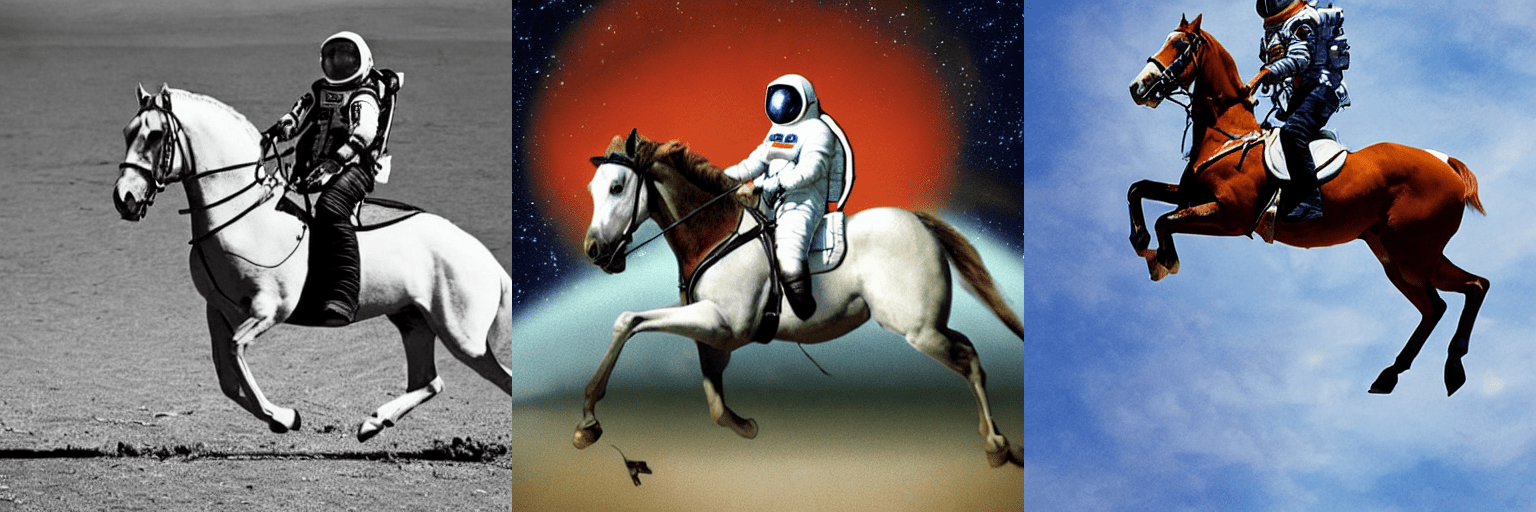By default, stable diffusion produces images of 512 × 512 pixels. It's very easy to override the default using the height and width arguments to create rectangular images in portrait or landscape ratios.

When choosing image sizes, we advise the following:

• Make sure height and width are both multiples of 8.
• Going below 512 might result in lower quality images.
• Going over 512 in both directions will repeat image areas (global coherence is lost).
• The best way to create non-square images is to use 512 in one dimension, and a value larger than that in the other one.

Let's run an example:

prompt = "a photograph of an astronaut riding a horse"
image = pipe(prompt, height=512, width=768).images

# you can save the image with
# image.save(f"astronaut_rides_horse.png")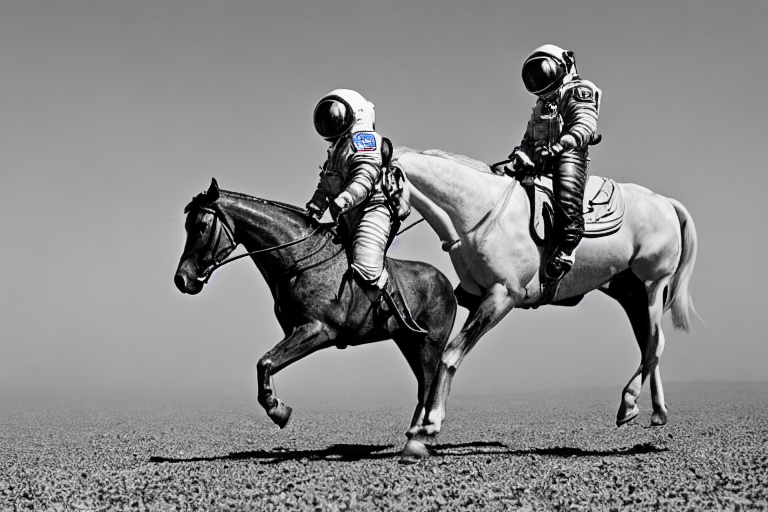## How does Stable Diffusion work?

Having seen the high-quality images that stable diffusion can produce, let's try to understand a bit better how the model functions.

Stable Diffusion is based on a particular type of diffusion model called Latent Diffusion, proposed in High-Resolution Image Synthesis with Latent Diffusion Models.

Generally speaking, diffusion models are machine learning systems that are trained to denoise random Gaussian noise step by step, to get to a sample of interest, such as an image. For a more detailed overview of how they work, check this colab.

Diffusion models have shown to achieve state-of-the-art results for generating image data. But one downside of diffusion models is that the reverse denoising process is slow because of its repeated, sequential nature. In addition, these models consume a lot of memory because they operate in pixel space, which becomes huge when generating high-resolution images. Therefore, it is challenging to train these models and also use them for inference.

Latent diffusion can reduce the memory and compute complexity by applying the diffusion process over a lower dimensional latent space, instead of using the actual pixel space. This is the key difference between standard diffusion and latent diffusion models: in latent diffusion the model is trained to generate latent (compressed) representations of the images.

There are three main components in latent diffusion.

1. An autoencoder (VAE).
2. A U-Net.
3. A text-encoder, e.g. CLIP's Text Encoder.

1. The autoencoder (VAE)

The VAE model has two parts, an encoder and a decoder. The encoder is used to convert the image into a low dimensional latent representation, which will serve as the input to the U-Net model. The decoder, conversely, transforms the latent representation back into an image.

During latent diffusion training, the encoder is used to get the latent representations (latents) of the images for the forward diffusion process, which applies more and more noise at each step. During inference, the denoised latents generated by the reverse diffusion process are converted back into images using the VAE decoder. As we will see during inference we only need the VAE decoder.

2. The U-Net

The U-Net has an encoder part and a decoder part both comprised of ResNet blocks. The encoder compresses an image representation into a lower resolution image representation and the decoder decodes the lower resolution image representation back to the original higher resolution image representation that is supposedly less noisy. More specifically, the U-Net output predicts the noise residual which can be used to compute the predicted denoised image representation.

To prevent the U-Net from losing important information while downsampling, short-cut connections are usually added between the downsampling ResNets of the encoder to the upsampling ResNets of the decoder. Additionally, the stable diffusion U-Net is able to condition its output on text-embeddings via cross-attention layers. The cross-attention layers are added to both the encoder and decoder part of the U-Net usually between ResNet blocks.

3. The Text-encoder

The text-encoder is responsible for transforming the input prompt, e.g. "An astronaut riding a horse" into an embedding space that can be understood by the U-Net. It is usually a simple transformer-based encoder that maps a sequence of input tokens to a sequence of latent text-embeddings.

Inspired by Imagen, Stable Diffusion does not train the text-encoder during training and simply uses an CLIP's already trained text encoder, CLIPTextModel.

Why is latent diffusion fast and efficient?

Since latent diffusion operates on a low dimensional space, it greatly reduces the memory and compute requirements compared to pixel-space diffusion models. For example, the autoencoder used in Stable Diffusion has a reduction factor of 8. This means that an image of shape (3, 512, 512) becomes (3, 64, 64) in latent space, which requires 8 × 8 = 64 times less memory.

This is why it's possible to generate 512 × 512 images so quickly, even on 16GB Colab GPUs!

Stable Diffusion during inference

Putting it all together, let's now take a closer look at how the model works in inference by illustrating the logical flow.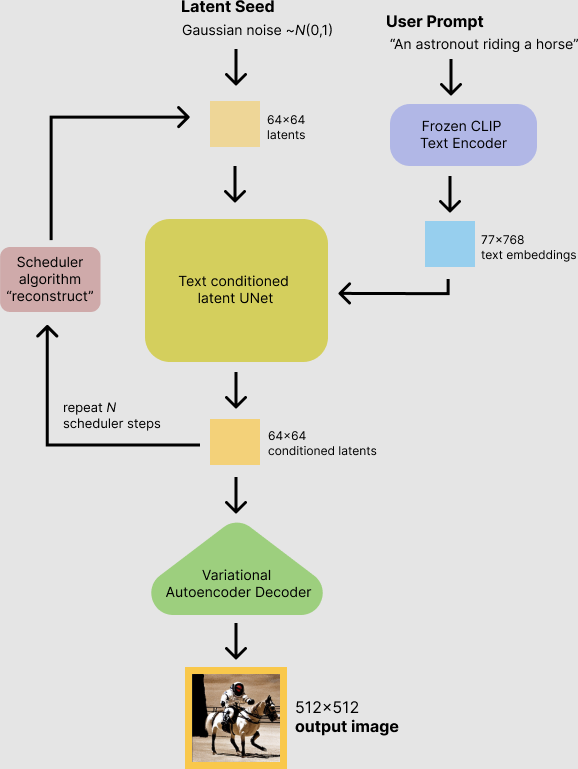The stable diffusion model takes both a latent seed and a text prompt as an input. The latent seed is then used to generate random latent image representations of size $64 \times 64$ where as the text prompt is transformed to text embeddings of size $77 \times 768$ via CLIP's text encoder.

Next the U-Net iteratively denoises the random latent image representations while being conditioned on the text embeddings. The output of the U-Net, being the noise residual, is used to compute a denoised latent image representation via a scheduler algorithm. Many different scheduler algorithms can be used for this computation, each having its pro- and cons. For Stable Diffusion, we recommend using one of:

Theory on how the scheduler algorithm function is out-of-scope for this notebook, but in short one should remember that they compute the predicted denoised image representation from the previous noise representation and the predicted noise residual. For more information, we recommend looking into Elucidating the Design Space of Diffusion-Based Generative Models

The denoising process is repeated ca. 50 times to step-by-step retrieve better latent image representations. Once complete, the latent image representation is decoded by the decoder part of the variational auto encoder.

After this brief introduction to Latent and Stable Diffusion, let's see how to make advanced use of 🤗 Hugging Face diffusers library!

## Writing your own inference pipeline

Finally, we show how you can create custom diffusion pipelines with diffusers. Writing a custom inference pipeline is an advanced use of the diffusers library that can be useful to switch out certain components, such as the VAE or scheduler explained above.

For example, we'll show how to use Stable Diffusion with a different scheduler, namely Katherine Crowson's K-LMS scheduler added in this PR.

The pre-trained model includes all the components required to setup a complete diffusion pipeline. They are stored in the following folders:

• text_encoder: Stable Diffusion uses CLIP, but other diffusion models may use other encoders such as BERT.
• tokenizer. It must match the one used by the text_encoder model.
• scheduler: The scheduling algorithm used to progressively add noise to the image during training.
• unet: The model used to generate the latent representation of the input.
• vae: Autoencoder module that we'll use to decode latent representations into real images.

We can load the components by referring to the folder they were saved, using the subfolder argument to from_pretrained.

from transformers import CLIPTextModel, CLIPTokenizer
from diffusers import AutoencoderKL, UNet2DConditionModel, PNDMScheduler

# 1. Load the autoencoder model which will be used to decode the latents into image space.
vae = AutoencoderKL.from_pretrained("CompVis/stable-diffusion-v1-4", subfolder="vae")

# 2. Load the tokenizer and text encoder to tokenize and encode the text.
tokenizer = CLIPTokenizer.from_pretrained("openai/clip-vit-large-patch14")
text_encoder = CLIPTextModel.from_pretrained("openai/clip-vit-large-patch14")

# 3. The UNet model for generating the latents.
unet = UNet2DConditionModel.from_pretrained("CompVis/stable-diffusion-v1-4", subfolder="unet")


from diffusers import LMSDiscreteScheduler

scheduler = LMSDiscreteScheduler(beta_start=0.00085, beta_end=0.012, beta_schedule="scaled_linear", num_train_timesteps=1000)


Next, let's move the models to GPU.

torch_device = "cuda"
vae.to(torch_device)
text_encoder.to(torch_device)
unet.to(torch_device)


We now define the parameters we'll use to generate images.

Note that guidance_scale is defined analog to the guidance weight w of equation (2) in the Imagen paper. guidance_scale == 1 corresponds to doing no classifier-free guidance. Here we set it to 7.5 as also done previously.

In contrast to the previous examples, we set num_inference_steps to 100 to get an even more defined image.

prompt = ["a photograph of an astronaut riding a horse"]

height = 512                        # default height of Stable Diffusion
width = 512                         # default width of Stable Diffusion

num_inference_steps = 100           # Number of denoising steps

guidance_scale = 7.5                # Scale for classifier-free guidance

generator = torch.manual_seed(0)    # Seed generator to create the inital latent noise

batch_size = len(prompt)


First, we get the text_embeddings for the passed prompt. These embeddings will be used to condition the UNet model and guide the image generation towards something that should resemble the input prompt.

text_input = tokenizer(prompt, padding="max_length", max_length=tokenizer.model_max_length, truncation=True, return_tensors="pt")

text_embeddings = text_encoder(text_input.input_ids.to(torch_device))


We'll also get the unconditional text embeddings for classifier-free guidance, which are just the embeddings for the padding token (empty text). They need to have the same shape as the conditional text_embeddings (batch_size and seq_length)

max_length = text_input.input_ids.shape[-1]
uncond_input = tokenizer(
[""] * batch_size, padding="max_length", max_length=max_length, return_tensors="pt"
)
uncond_embeddings = text_encoder(uncond_input.input_ids.to(torch_device))


For classifier-free guidance, we need to do two forward passes: one with the conditioned input (text_embeddings), and another with the unconditional embeddings (uncond_embeddings). In practice, we can concatenate both into a single batch to avoid doing two forward passes.

text_embeddings = torch.cat([uncond_embeddings, text_embeddings])


Next, we generate the initial random noise.

latents = torch.randn(
(batch_size, unet.in_channels, height // 8, width // 8),
generator=generator,
)
latents = latents.to(torch_device)


If we examine the latents at this stage we'll see their shape is torch.Size([1, 4, 64, 64]), much smaller than the image we want to generate. The model will transform this latent representation (pure noise) into a 512 × 512 image later on.

Next, we initialize the scheduler with our chosen num_inference_steps. This will compute the sigmas and exact time step values to be used during the denoising process.

scheduler.set_timesteps(num_inference_steps)


The K-LMS scheduler needs to multiply the latents by its sigma values. Let's do this here:

latents = latents * scheduler.init_noise_sigma


We are ready to write the denoising loop.

from tqdm.auto import tqdm

scheduler.set_timesteps(num_inference_steps)

for t in tqdm(scheduler.timesteps):
# expand the latents if we are doing classifier-free guidance to avoid doing two forward passes.
latent_model_input = torch.cat([latents] * 2)

latent_model_input = scheduler.scale_model_input(latent_model_input, timestep=t)

# predict the noise residual
noise_pred = unet(latent_model_input, t, encoder_hidden_states=text_embeddings).sample

# perform guidance
noise_pred_uncond, noise_pred_text = noise_pred.chunk(2)
noise_pred = noise_pred_uncond + guidance_scale * (noise_pred_text - noise_pred_uncond)

# compute the previous noisy sample x_t -> x_t-1
latents = scheduler.step(noise_pred, t, latents).prev_sample


We now use the vae to decode the generated latents back into the image.

# scale and decode the image latents with vae
latents = 1 / 0.18215 * latents
image = vae.decode(latents).sample


And finally, let's convert the image to PIL so we can display or save it.

image = (image / 2 + 0.5).clamp(0, 1)
image = image.detach().cpu().permute(0, 2, 3, 1).numpy()
images = (image * 255).round().astype("uint8")
pil_images = [Image.fromarray(image) for image in images]
pil_images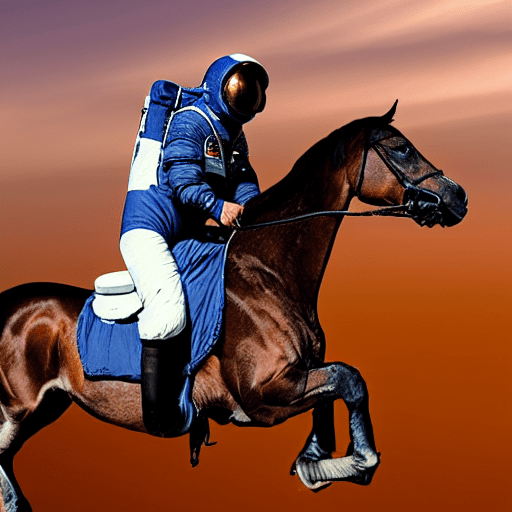We've gone from the basic use of Stable Diffusion using 🤗 Hugging Face Diffusers to more advanced uses of the library, and we tried to introduce all the pieces in a modern diffusion system. If you liked this topic and want to learn more, we recommend the following resources: